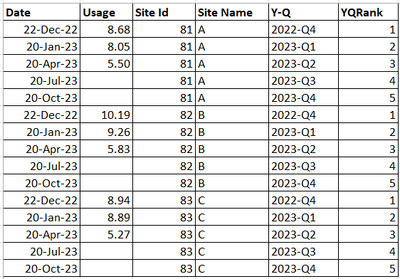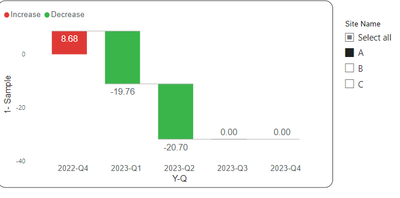cancel
Showing results for
Did you mean:

Fabric is Generally Available. Browse Fabric Presentations. Work towards your Fabric certification with the Cloud Skills Challenge.Frequent Visitor

## Waterfall chart: Current quarter - Previous Quarter logic is not working

Hi,

I'm trying to create a waterfall chart that shows a Quarter in X-axis and the raise and fall of usage in the Yaxis,

first quarter should show the actual value of that quarter and from the next quarter onwards it should show the current - previous

Table I'm using = Sample

 Date Usage Site Id Site Name Y-Q YQRank 22-Dec-22 8.68 81 A 2022-Q4 1 20-Jan-23 8.05 81 A 2023-Q1 2 20-Apr-23 5.50 81 A 2023-Q2 3 20-Jul-23 81 A 2023-Q3 4 20-Oct-23 81 A 2023-Q4 5 22-Dec-22 10.19 82 B 2022-Q4 1 20-Jan-23 9.26 82 B 2023-Q1 2 20-Apr-23 5.83 82 B 2023-Q2 3 20-Jul-23 82 B 2023-Q3 4 20-Oct-23 82 B 2023-Q4 5 22-Dec-22 8.94 83 C 2022-Q4 1 20-Jan-23 8.89 83 C 2023-Q1 2 20-Apr-23 5.27 83 C 2023-Q2 3 20-Jul-23 83 C 2023-Q3 4 20-Oct-23 83 C 2023-Q4 5The Dax measure which I'm using is working fine when I choose all site names, but it is not working fine when I choose a particular site (Ex: A) form the slicer.

Measure I'm currently using

Measure =
VAR __Current = sum('Sample'[Usage])
VAR __CurrentQR = MAX('Sample'[YQRank])
VAR __Previous = SUMX(FILTER(ALL('Sample'),[YQRank] = __CurrentQR - 1), [Usage])
VAR __Result = IF(__Current=BLANK(),"0",__Current - __Previous)
RETURN
__Result

Working fine when I choose all Site NamesWhen I select Only one site name for example 'A', It is giving wrong output as shown below.Expected Result

When I select only 'A' The values should be
2022-Q4 = 8.68
2023-Q1 = -0.63  ----> (8.05-8.68)
2023-Q2 = -2.55  ----> (5.50-8.05)

1 ACCEPTED SOLUTIONHelper I

Hi Veena,

Try with below measure

Measure =
VAR __Current = sum('Sample'[Usage])
VAR __Table = FILTER(ALLSELECTED('Sample'),'Sample'[Site Name] = "A") // since we are not using calculate function here, so first we need to filterout the table with required category and keep it in avariable
VAR __CurrentQR = MAX('Sample'[YQRank])
VAR __Previous = SUMX(FILTER(__Table,[YQRank] = __CurrentQR - 1), [Usage])
VAR __Result = IF(__Current=BLANK(),"0",__Current - __Previous)
RETURN
__Result

To make it dynamic based on slicer selection (single/multiple/all ) use below measure
Measure 1 =
VAR __Site = VALUES('Sample'[Site Name])
VAR __Current = sum('Sample'[Usage])
VAR __Table = FILTER(ALLSELECTED('Sample'),'Sample'[Site Name]in __Site)
VAR __CurrentQR = MAX('Sample'[YQRank])
VAR __Previous = SUMX(FILTER(__Table,[YQRank] = __CurrentQR - 1), [Usage])
VAR __Result = IF(__Current=BLANK(),"0",__Current - __Previous)
RETURN
__Result
3 REPLIES 3Helper I

Try with below measure

Measure =
VAR __Current = sum('Sample'[Usage])
VAR __Table = FILTER(ALLSELECTED('Sample'),'Sample'[Site Name] = "A") // since we are not using calculate function here, so first we need to filterout the table with required category and keep it in avariable
VAR __CurrentQR = MAX('Sample'[YQRank])
VAR __Previous = SUMX(FILTER(__Table,[YQRank] = __CurrentQR - 1), [Usage])
VAR __Result = IF(__Current=BLANK(),"0",__Current - __Previous)
RETURN
__Result

To make it dynamic based on slicer selection (single/multiple/all ) use below measure
Measure 1 =
VAR __Site = VALUES('Sample'[Site Name])
VAR __Current = sum('Sample'[Usage])
VAR __Table = FILTER(ALLSELECTED('Sample'),'Sample'[Site Name]in __Site)
VAR __CurrentQR = MAX('Sample'[YQRank])
VAR __Previous = SUMX(FILTER(__Table,[YQRank] = __CurrentQR - 1), [Usage])
VAR __Result = IF(__Current=BLANK(),"0",__Current - __Previous)
RETURN
__ResultHelper I

Hi Veena,

Try with below measure

Measure =
VAR __Current = sum('Sample'[Usage])
VAR __Table = FILTER(ALLSELECTED('Sample'),'Sample'[Site Name] = "A") // since we are not using calculate function here, so first we need to filterout the table with required category and keep it in avariable
VAR __CurrentQR = MAX('Sample'[YQRank])
VAR __Previous = SUMX(FILTER(__Table,[YQRank] = __CurrentQR - 1), [Usage])
VAR __Result = IF(__Current=BLANK(),"0",__Current - __Previous)
RETURN
__Result

To make it dynamic based on slicer selection (single/multiple/all ) use below measure
Measure 1 =
VAR __Site = VALUES('Sample'[Site Name])
VAR __Current = sum('Sample'[Usage])
VAR __Table = FILTER(ALLSELECTED('Sample'),'Sample'[Site Name]in __Site)
VAR __CurrentQR = MAX('Sample'[YQRank])
VAR __Previous = SUMX(FILTER(__Table,[YQRank] = __CurrentQR - 1), [Usage])
VAR __Result = IF(__Current=BLANK(),"0",__Current - __Previous)
RETURN
__ResultFrequent Visitor

Hi,

Thanks for the reply, it is working as expected. that was a great help.Announcements#### Power BI Monthly Update - November 2023

Check out the November 2023 Power BI update to learn about new features.#### Fabric Community News unified experience

Read the latest Fabric Community announcements, including updates on Power BI, Synapse, Data Factory and Data Activator.#### Exclusive opportunity for Women!

Join us for a free, hands-on Microsoft workshop led by women trainers for women where you will learn how to build a Dashboard in a Day!#### The largest Power BI and Fabric virtual conference

130+ sessions, 130+ speakers, Product managers, MVPs, and experts. All about Power BI and Fabric. Attend online or watch the recordings.Top Solution Authors
Top Kudoed Authors
Users online (4,505)